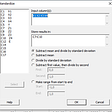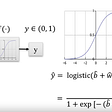# Introduction

While dealing with Linear Regression we can have multiple lines for different values of slopes and intercepts. But the main question that arises is which of those lines actually represents the right relationship between the X and Y and in order to find that we can use the Mean Squared Error or MSE as the parameter. For linear regression, this MSE is nothing but the Cost Function.

Mean Squared Error is the sum of the squared differences between the prediction and true value. And the output is a single number representing the cost. So the line with the minimum cost function or MSE represents the relationship between X and Y in the best possible manner. And once we have the slope and intercept of the line which gives the least error, we can use that line to predict Y.

As we know that any line can be represented by two parameters- slope(β) and intercept(b).

And once we have a line we can always calculate the errors(also known as cost or loss) which this line would have from the underlying data point and the idea is to find the line which gives us the least error. This basically becomes an optimization problem. Let’s go through an exercise where you’ll see what is the error for various values of β and B and then the question is how do we find the most optimum values of these two parameters.

# Let’s Begin!

I’ll start by importing the necessary libraries which are matplotlib, pandas and scikit learn to calculate the error:

And I have created a data set for Experience and Salary. For that, I’ve created a list and then just simply converted it to a Pandas Dataframe using pd.DataFrame():

You can see the first five rows of our dataset.

Let us now explore the dataset by exploring the relationship between salary and experience. Let’s plot this using Matplotlib:

You can see a linear relationship between experience and salary. And this is what we expected. So let’s now start by plotting the lines by using various values of β and b.

To start with let me take β = 0.1 and b = 1.1 and what I’m going to do is create a line with these two parameters and plot it over the scatter plot. Now, I take values and then apply this relationship to create each of these lines and then I plot this over the above-created scatter plot.

We have this line for beta = 0.1 and b = 1.1 and the MSE for this line is 2.69. Now as you can see the slope of the line is very less so I would want to actually try out a higher slope so instead of beta = 0.1 let me change this to beta = 1.5 :

This is the line for beta = 1.5 and b = 1.1 and the MSE for this line is 6.40. You can see a better slope but it is probably more than what we actually want. So the right value might be somewhere in between 0.1 and1.5, so let’s try beta = 0.8:

This is the line with beta = 0.8 and b = 1.1 and we can see that the mean squared error has come down to 0.336

We have tried 3 different lines with each of these having different MSE; the first one had 2.69, the second had 6.4 and the third one had 0.33. Now we can go on and try this for various values of beta. So now we can try this with various values of Beta and see what is the relationship between beta and mean squared error(MSE), for a fixed value intercept i.e b. So we’re not changing b.

# Computing Cost Function over a range of values of Beta

So let’s create a function which I am calling as Error and what this function does is for a given value beta it is basically giving me what is the MSE for these data points. Here b is fixed and I am trying different values of Beta.

Now I am trying all values of Beta between 0 to 1.5 with an increment of 0.01 and I’ll then append everything to a list:

# Visualizing cost with respect to Beta

When this is done, just convert this into this is the Data Frame:

What this data frame is showing that for a value of Beta which is 0.00 the cost or MSE we’re getting is 3.72, similarly for beta = 0.04, we are getting cost = 3.29. Let’s quickly visualize this:

This is the plot that we get. So as you can see the value of cost at 0 was around 3.72, so that is the starting value. After that the error goes down with the increasing value of Beta, reaches a minimum, and then it starts increasing.

Now the question is given that we know this relationship, what are the values of beta and b for which we can find out this particular location where my cost is minimum.

Now what happens when you have two parameters, I mean right now we assumed b to be 0.1 but actually what we want to do is change it with respect to b as well as beta and in this particular situation this is the curve which we get:

So again, this is just a 3D plot, so you have two dimensions but it will again have a minimum value and the idea would be to find the minimum value. But you cannot do this iteratively in the way we did so far. So what we need to find is a smarter way in which we can find this minimum value and that is where the Gradient Descent technique comes into play.

--

--

--

Love podcasts or audiobooks? Learn on the go with our new app.

## Data Science👨‍💻: Data Preprocessing with Orange Tool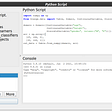## Using Box-Cox to Achieve Multivariate Normality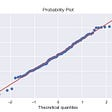## Can LSTM be used to Forecast the Number of Sunspots?## Geo Maps using SAP Analytics Cloud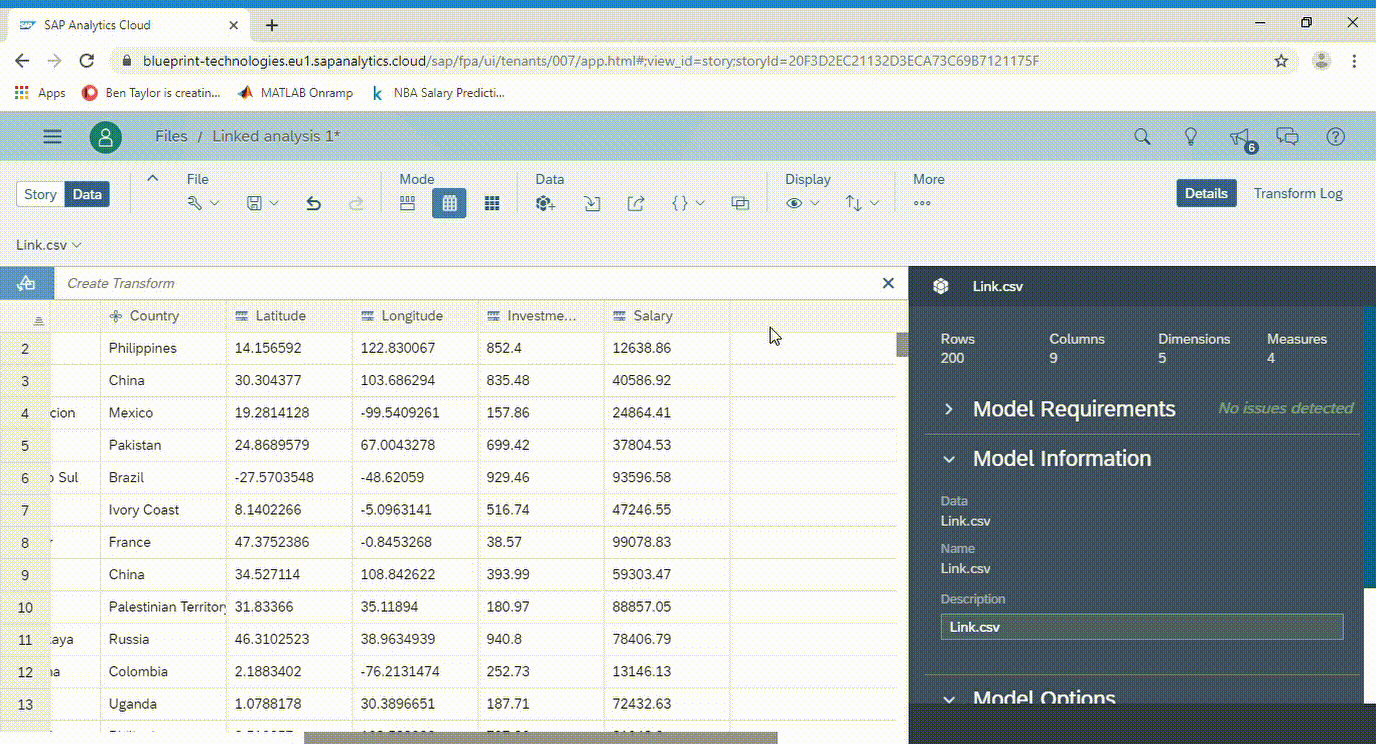## DTC 206 Assignment 3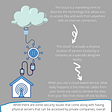## Data Classification explained## Importance of Knowledge in the 21st Century## Entropy : An essential measurement in machine learning.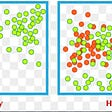## Have you ever been in doing the analysis, having data that do not meet the assumption of…